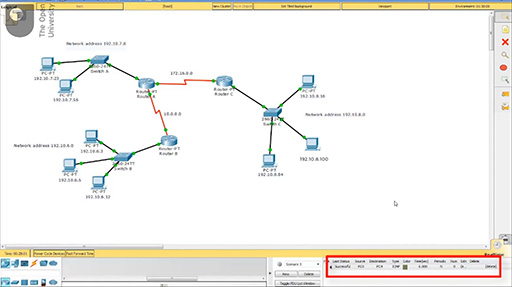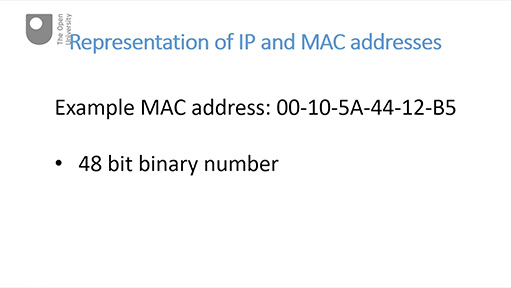Discovering computer networks: hands on in the Open Networking Lab

Start this free course now. Just create an account and sign in. Enrol and complete the course for a free statement of participation or digital badge if available.

Free course

# 7.3 Representation of IP and MAC addresses

This part explains how the binary numbers of an IP address and a MAC address are represented to be more readable for humans. There are two videos in this part – each video is followed by an activity.

Watch the video below, which is about 4 minutes long.

Note that at 00:45 the speaker says ‘provides nearly 4 million 3 hundred different addresses’, but meant to say ‘provides nearly 4.3 billion addresses’.Skip transcript

#### Transcript

Hello. Now you’re familiar with the idea of representing numbers with different number bases, we’ll have a closer look at how network addresses are represented. In this video I’ll look at IP addresses, specifically IP version 4 addresses and in the next video I’ll look at MAC addresses.

Remember we started off this session in Part 1 with a reminder of what an IP address and a MAC address look like.

Computers can only recognise these addresses as a series of zeros and ones, but humans find long strings of zeros and ones difficult to read and interpret so this is why we represent them in this way.

An IPv4 address is in fact a 32-bit binary number which, as you know from Part 1, provides nearly 4 million 3 hundred different addresses.

But let’s just focus on the IP address. To a computer, this would look like this.

You can see how difficult it is to read this number, so for convenience I’ll show the 32 binary numbers in groups of four bits.

When representing an IP address as a decimal number, we actually deal with these groups in pairs. This gives us four groups of eight bits. A group of 8 bits is often referred to as an octet. So here we have four octets. What’s the highest decimal number we can represent using 8 bits? Well, the highest 8-bit number has a 1 in every place. What would this be in decimal? We could go for the easy way of answering this question which would be to use a binary-to-decimal converter – maybe one of those I showed you in Part 2 of this session.

Or we could calculate it ourselves using the weighting system I discussed in Part 1 of this session. Because there’s a 1 in each position this would result in adding together 2 to the seven, 2 to the six, 2 to the five, 2 to the four, 2 to the three, 2 to the two, 2 to the one and 2 to the zero giving 255.

But there’s a quicker way than this. 8 binary bits enables us to represent 2 to the eight different decimal numbers. That equals 256 in total. One of these numbers will, of course be a zero, making it 255 as the highest decimal number we can represent with a group of 8 bits.

So that’s 2 to the eight minus 1 equals 255. So 255 is the highest decimal number we can represent using 8 binary bits.

This provides us with a neat and easy way of working out the highest decimal number we can represent with any given number of binary bits. It will be 2 to the n, minus 1, where n is the number of binary bits.

But I’ve digressed – a little bit! Let’s go back to that IP address.

The final step in translating the 32 binary bits into the dotted decimal equivalent is to convert each of these four octets into a decimal number. These are then shown together separated by dots as you can see in this example IP address here.

This representation is known as dotted decimal.

In the next video we’ll look at the representation of a MAC address.

End transcript

Interactive feature not available in single page view (see it in standard view).

## Activity 5 Test yourself

5 minutes

• What is the highest decimal number that could be represented by 10 binary symbols? (Hint: use the formula introduced in the video.)

• The formula is 2n − 1. You need to substitute a 10 for the n in the exponent. This gives 210 − 1 = 1024 − 1 = 1023.

Watch the video below, which is about 3 minutes long.Skip transcript

#### Transcript

Now we’ll turn our attention to the MAC address.

A MAC address (sometimes called the hardware address or physical address) is also a string of binary numbers. A MAC address is a 48-bit binary number– 16 bits longer than an IPv4 address – giving it 6 octets rather than the 4 octets in an IPv4 address.

Though these could also be represented in dotted decimal, it’s more efficient to use a different number base: base 16 (hexadecimal). Using hexadecimal it’s possible to represent an octet as a group of two symbols rather than the group of three symbols required by decimal.

Hexadecimal follows the same rules as the previous number bases we’ve discussed, but this time the weightings are made in multiples of 16.

Of course, we don’t have 16 different number symbols, so we borrow some from the alphabet to make up the difference. Here are the symbols and their decimal equivalents.

So you can see that when we get to the hexadecimal equivalent of 10 we’ve borrowed an A from the alphabet and so on. Looking at the example MAC address given earlier, you’ll see some of these letter symbols.

So what is the highest decimal number we could represent with a group of two hexadecimal symbols? Well, here’s where that formula comes in again: the one I gave you to work out the highest decimal number that can be represented using a group of binary bits.

But here we’re using hexadecimal symbols not binary, so we substitute 16 for 2.

And the question I asked was ‘What’s the highest decimal number we could represent with a group of two hexadecimal symbols?’ so we substitute 2 for n in the exponent, giving us 16 squared minus 1. Result: 256 minus 1 equals 255.

So whereas we needed to use 8 binary bits to represent a decimal number of up to 255, we only need two hexadecimal symbols to do the same job. Or, to put it another way, a binary octet (8 bits) can be represented either by three decimal symbols or two hexadecimal symbols. Hexadecimal is therefore more efficient, especially when representing large numbers such as MAC addresses.

So a MAC address consists of six pairs of hexadecimal numbers, usually separated by a hyphen but sometimes you will see them separated by a colon. Each pair represents one binary octet.

You may be wondering why, if it’s more efficient, we don’t express IP addresses in hexadecimal too. I’ve wondered that myself. I think the answer is that it just comes down to convention.

So, you’ve seen that an IP address is a 32-bit binary number that we represent as four 3-digit decimal numbers, separated by dots, and a MAC address is a 48-bit binary number that we represent as six 2-digit hexadecimal numbers, usually separated by hyphens.

End transcript

Interactive feature not available in single page view (see it in standard view).

## Activity 6 Test yourself

5 minutes

• 1. What is the decimal equivalent of hexadecimal D?

• D is the hexadecimal equivalent of decimal 13.

• 2. Use the Windows calculator or an online converter to ascertain the highest possible MAC address. (Hint: it’s easier to enter this in hexadecimal rather than 48 binary bits.)

• You know that 48 binary bits of a MAC address are represented by 6 pairs of hexadecimal numbers. The highest hexadecimal number is F so you will need to enter F twelve times: FF FF FF FF FF FF. This will produce the decimal equivalent 281,474,976,710,655.

• 3. If instead the highest MAC address was represented in dotted decimal, what would it be? (Hint: the highest MAC address in binary has a 1 in every place.)

• The highest binary number for any given number of bits would have a 1 in every place. 48 bits would be 6 octets and the highest decimal number that can be represented by a binary octet is 255. Therefore, if this were to be represented in dotted decimal it would be 255.255.255.255.255.255.

Note: in many other contexts 8 bits are referred to as a byte, but in the context of MAC addressing it is more normal to refer to 8 bits as an octet.

OPNL_1